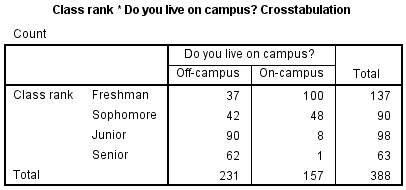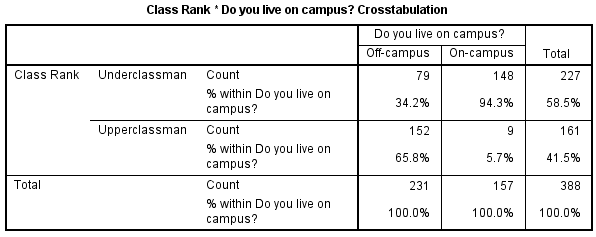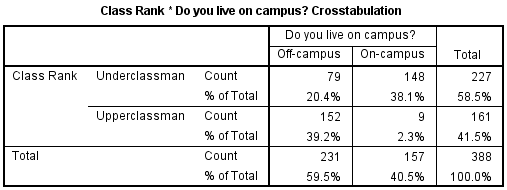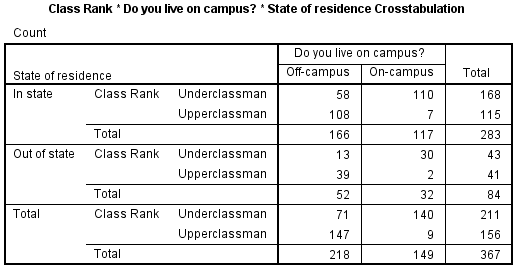# SPSS Tutorials: Crosstabs

The Crosstabs procedure is used to create contingency tables, which describe the interaction between two categorical variables.

## Crosstabs

To describe a single categorical variable, we use frequency tables. To describe the relationship between two categorical variables, we use a special type of table called a cross-tabulation (or "crosstab" for short). In a cross-tabulation, the categories of one variable determine the rows of the table, and the categories of the other variable determine the columns. The cells of the table contain the number of times that a particular combination of categories occurred. The "edges" (or "margins") of the table typically contain the total number of observations for that category.

This type of table is also known as a:

• Crosstab.
• Two-way table.
• Contingency table.

## Describing a Crosstab

The dimensions of the crosstab refer to the number of rows and columns in the table. (The "total" row/column are not included.) The table dimensions are reported as as RxC, where R is the number of categories for the row variable, and C is the number of categories for the column variable.

Additionally, a "square" crosstab is one in which the row and column variables have the same number of categories. Tables of dimensions 2x2, 3x3, 4x4, etc. are all square crosstabs.

#### Example 1: A "square" table (2x2)• Row variable: Gender (2 categories: male, female)
• Column variable: Alcohol (2 categories: no, yes)
• Table dimension: 2x2 (square)

#### Example 2: A "long" table (4x2)• Row variable: Class Rank (4 categories: freshman, sophomore, junior, senior)
• Column variable: Gender (2 categories: male, female)
• Table dimension: 4x2

#### Example 3: A "wide" table (2x3)• Row variable: Gender (2 categories: male, female)
• Column variable: Smoking (3 categories: never smoked, past smoker, current smoker)
• Table dimension: 2x3

## Understanding Row, Column, and Total Percents

A typical 2x2 crosstab has the following construction:

Column 1 Column 2 Row totals
Row 1 a b a + b
Row 2 c d c + d
Column totals a + c b + d a + b + c + d

The letters a, b, c, and d represent what are called cell counts.

• a is the number of observations corresponding to Row 1 AND Column 1.
• b is the number of observations corresponding to Row 1 AND Column 2.
• c is the number of observations corresponding to Row 2 AND Column 1.
• d is the number of observations corresponding to Row 2 AND Column 2.

By adding a, b, c, and d, we can determine the total number of observations in each category, and in the table overall.

• Row sum of row 1 (i.e., total number of observations in Row 1): a + b
• Row sum of row 2 (i.e., total number of observations in Row 2): c + d
• Column sum of column 1 (i.e., total number of observations in Column 1): a + c
• Column sum of column 2 (i.e., total number of observations in Column 2): b + d
• Total sum (i.e., total number of observations in the table): n = a + b + c + d

The row sums and column sums are sometimes referred to as marginal frequencies. Note that if you were to make frequency tables for your row variable and your column variable, the frequency table should match the values for the row totals and column totals, respectively.

When you are describing the composition of your sample, it is often useful to refer to the proportion of the row or column that fell within a particular category. This can be achieved by computing the row percentages or column percentages.

Column 1 Column 2 Row totals
Formulas for computing row percentages

Row 1

Row 1 %

a

a / (a + b)

b

b / (a + b)

a + b

(a + b) / (a+b) = 100%

Row 2

Row 2 %

c

c / (c + d)

d

d / (c + d)

c + d

(c + d) / (c + d) = 100%

Column totals

% of total

a + c

(a + c) / (a + b + c + d)

b + d

(b + d) / (a + b + c + d)

a + b + c + d

(a + b + c + d) / (a + b + c + d) = 100%

Notice that when computing row percentages, the denominators for cells a, b, c, d are determined by the row sums (here, a + b and c + d). This implies that the percentages in the "row totals" column must equal 100%.

Column 1 Column 2 Row totals
Formulas for computing column percentages

Row 1

Column 1 %

a

a / (a + c)

b

b / (b + d)

a + b

(a + b) / (a + b + c + d)

Row 2

Column 2 %

c

c / (a + c)

d

d / (b + d)

c + d

(c + d) / (a + b + c + d)

Column totals

Percentage %

a + c

(a + c) / (a + c) = 100%

b + d

(b + d) / (b + d) = 100%

a + b + c + d

(a + b + c + d) / (a + b + c + d) = 100%

Notice that when computing column percentages, the denominators for cells a, b, c, d are determined by the column sums (here, a + c and b + d). This implies that the percentages in the "column totals" row must equal 100%.

Column 1 Column 2 Row totals
Formulas for computing total percentages

Row 1

% of total

a

a / (a + b + c + d)

b

b / (a + b + c + d)

a + b

(a + b) / (a + b + c + d)

Row 2

% of total

c

c / (a + b + c + d)

d

d / (a + b + c + d)

c + d

(c + d) / (a + b + c + d)

Column totals

% of total

a + c

(a + c) / (a + b + c + d)

b + d

(b + d) / (a + b + c + d)

a + b + c + d

(a + b + c + d) / (a + b + c + d) = 100%

Notice that when total percentages are computed, the denominators for all of the computations are equal to the total number of observations in the table, i.e. a + b + c + d.

## Data Set-Up and Requirements

### Data Requirements

Your data must meet the following requirements:

1. Two categorical variables.
2. Two or more categories (groups) for each variable.

### Data Set-Up

The categorical variables in your SPSS dataset can be numeric or string, and their measurement level can be defined as nominal, ordinal, or scale. However, crosstabs should only be used when there are a limited number of categories.

Note that in most cases, the row and column variables in a crosstab can be used interchangeably. The choice of row/column variable is usually dictated by space requirements or interpretation of the results.

## Create a Crosstab in SPSS

To create a crosstab, click Analyze > Descriptive Statistics > Crosstabs.A Row(s): One or more variables to use in the rows of the crosstab(s). You must enter at least one Row variable.

B Column(s): One or more variables to use in the columns of the crosstab(s). You must enter at least one Column variable.

Also note that if you specify one row variable and two or more column variables, SPSS will print crosstabs for each pairing of the row variable with the column variables. The same is true if you have one column variable and two or more row variables, or if you have multiple row and column variables.

C Layer: An optional "stratification" variable. When a layer variable is specified, the crosstab between the Row and Column variable(s) will be created at each level of the layer variable. You can have multiple layers of variables by specifying the first layer variable and then clicking Next to specify the second layer variable. Alternatively, you can try out multiple variables as single layers at a time by putting them all in the Layer 1 of 1 box.

D Statistics: Opens the Crosstabs: Statistics window, which contains fifteen different inferential statistics for comparing categorical variables. (These statistics will be covered in detail in a later tutorial.)E Cells: Opens the Crosstabs: Cell Display window, which controls which output is displayed in each cell of the crosstab.F Format: Opens the Crosstabs: Table Format window, which specifies how the rows of the table are sorted.## Example: Summarizing the Relationships of Three Categorical Variables

### Problem Statement

Some universities in the United States require that freshmen live in the on-campus dormitories during their first year, with exceptions for students whose families live within a certain radius of campus. That is, certain freshmen whose families live close enough to campus are permitted to live off-campus. After completing their first or second year of school, students living in the dorms may choose to move into an off-campus apartment. How prevalent is this pattern?

In the sample dataset, there are several variables relating to this question:

• Rank - Class rank (Freshmen, Sophomore, Junior, Senior)
• LiveOnCampus - Do you live on campus? (Yes/No)
• State - Are you an in-state or out-of-state student? (In State, Out of state)
• State_Residency - State residency, converted from string to numeric so that missing values are correctly identified (See the Automatic Recode tutorial)

Let's use different aspects of the Crosstabs procedure to investigate the relationship between class rank and living on campus.

### Part 1 - Simple Crosstabs

Using the sample data, let's make crosstab of the variables Rank and LiveOnCampus. Let the row variable be Rank, and the column variable be LiveOnCampus.

#### Running the Procedure

##### Using the Crosstabs Dialog Window
1. Open the Crosstabs window (Analyze > Descriptive Statistics > Crosstabs).
2. Select Rank as the row variable, and LiveOnCampus as the column variable.
3. Click OK.
##### Using Syntax
CROSSTABS
/TABLES=Rank BY LiveOnCampus
/FORMAT=AVALUE TABLES
/CELLS=COUNT
/COUNT ROUND CELL.

#### OutputThe Case Processing Summary tells us what proportion of the observations had nonmissing values for both Rank and LiveOnCampus. In this sample, there were 47 cases that had a missing value for Rank, LiveOnCampus, or for both Rank and LiveOnCampus.

The second table (here, Class Rank * Do you live on campus? Crosstabulation) contains the crosstab. We can quickly observe information about the interaction of these two variables:

• Many more freshmen lived on-campus (100) than off-campus (37)
• About an equal number of sophomores lived off-campus (42) versus on-campus (48)
• Far more juniors lived off-campus (90) than on-campus (8)
• Only one (1) senior lived on campus; the rest lived off-campus (62)

Note the margins of the crosstab (i.e., the "total" row and column) give us the same information that we would get from frequency tables of Rank and LiveOnCampus, respectively:

• The sample had 137 freshmen, 90 sophomores, 98 juniors, and 63 seniors
• There were 231 individuals who lived off-campus, and 157 individuals lived on-campus

### Part 2 - Row, column, and total percentages

Let's build on the table shown in Example 1 by adding row, column, and total percentages. For simplicity's sake, let's switch out the variable Rank (which has four categories) with the variable RankUpperUnder (which has two categories).

#### Running the Procedure

##### Using the Crosstabs Dialog Window
1. Reopen the Crosstabs window (Analyze > Descriptive Statistics > Crosstabs).
2. In the Row box, replace variable Rank with RankUpperUnder.
3. Click Cells. In the Percentages area, check off Row, Column, and Total percentages. (In the following examples, we will be showing each of these one at a time for ease of reading.) Click Continue.
4. Click OK to run.
##### Using Syntax
CROSSTABS
/TABLES=RankUpperUnder BY LiveOnCampus
/FORMAT=AVALUE TABLES
/CELLS=COUNT ROW COLUMN TOTAL
/COUNT ROUND CELL.

#### Output

##### Row percents

If the row variable is RankUpperUnder and the column variable is LiveOnCampus, then the row percentages will tell us what percentage of the upperclassmen or what percentage of the underclassmen live on campus. That is, variable RankUpperUnder will determine the denominator of the percentage computations.• The proportion of underclassmen who live off campus is 34.8%, or 79/227.
• The proportion of underclassmen who live on campus is 65.2%, or 148/226.
• The proportion of upperclassmen who live off campus is 94.4%, or 152/161.
• The proportion of upperclassmen who live on campus is 5.6%, or 9/161.
##### Column percents

If the row variable is RankUpperUnder and the column variable is LiveOnCampus, then the column percentages will tell us what percentage of the individuals who live on campus are upper or underclassmen. That is, variable LiveOnCampus will determine the denominator of the percentage computations.• The proportion of individuals living off campus who are underclassmen is 34.2%, or 79/231.
• The proportion of individuals living off campus who are upperclassmen is 65.8%, or 152/231.
• The proportion of individuals living on campus who are underclassmen is 94.3%, or 148/157.
• The proportion of individuals living on campus who are upperclassmen is 5.7%, or 9/157.
##### Total percents

If the row variable is RankUpperUnder and the column variable is LiveOnCampus, then the total percentage tells us what proportion of the total is within each combination of RankUpperUnder and LiveOnCampus. That is, the overall table size determines the denominator of the percentage computations.• Underclassmen living off campus make up 20.4% of the sample (79/388).
• Underclassmen living on campus make up 38.1% of the sample (148/388).
• Upperclassmen living off campus make up 39.2% of the sample (152/388).
• Upperclassmen living on campus make up 2.3% of the sample (9/388).

### Part 3 - Crosstabs with Layer Variable

Let's modify our analysis slightly by taking into account the students' state of residence (in-state or out-of-state). Here, we will be working with three categorical variables: RankUpperUnder, LiveOnCampus, and State_Residency.

In this example, we want to create a crosstab of RankUpperUnder by LiveOnCampus, with variable State_Residency acting as a strata, or layer variable.

#### Running the Procedure

##### Using the Crosstabs Dialog Window
1. Open the Crosstabs dialog (Analyze > Descriptive Statistics > Crosstabs).
2. Select RankUpperUnder as the row variable, and LiveOnCampus as the column variable.
3. Select State_Residency as the layer variable.
4. You may want to go back to the Cells options and turn off the row, column, and total percentages if you have just run the previous example.
5. Click OK.
##### Syntax
CROSSTABS
/TABLES=RankUpperUnder BY LiveOnCampus BY State_Residency
/FORMAT=AVALUE TABLES
/CELLS=COUNT
/COUNT ROUND CELL.

#### Output

Again, the Crosstabs output includes the boxes Case Processing Summary and the crosstabulation itself.Notice that after including the layer variable State Residency, the number of valid cases we have to work with has dropped from 388 to 367. This is because the crosstab requires nonmissing values for all three variables: row, column, and layer.The layered crosstab shows the individual Rank by Campus tables within each level of State Residency. Some observations we can draw from this table include:

• A slightly higher proportion of out-of-state underclassmen live on campus (30/43) than do in-state underclassmen (110/168).
• There were about equal numbers of out-of-state upper and underclassmen; for in-state students, the underclassmen outnumbered the upperclassmen.
• Of the nine upperclassmen living on-campus, only two were from out of state.